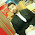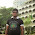## Friday, March 29, 2013

### Transformers Objective Questions: Part-7

 During light loads, the transformer efficiency is low because
A. Secondary output is low
B. Transformer losses are high
C. Fixed loss is high in proportion to the output
D. Cu loss is small

 The open circuit test is carried out in a transformer to find the
A. Cu loss
B. Core loss
C. Total loss
D. Insulation resistance

 The equivalent resistance of the primary of the transformer having K = 5, R1 = 0.1 ohm when referred to secondary becomes .....ohm
A. 0.5
B. 0.02
C. 0.004
D. 2.5

 A transformer has negative voltage regulation when its load power factor is
A. Zero
B. Unity
D. Lagging

 A transformer has 1000 primary turns, connected to 250V ac supply. To get 400V secondary voltage the no of secondary turns should be
A. 1600
B. 250
C. 400
D. 1250

 The iron loss of the transformer is negligible during short circuit test because
A. The entire input is just sufficient to meet Cu losses only
B. Flux produced is a small fraction of the normal flux
C. Iron core becomes fully saturated
D. Supply frequency is held constant

 In operating a 400hz transformer at 50Hz
A. Only voltage is reduced in the same proportion as the frequency
B. Only KVA rating is reduced in the same proportion as the frequency
C. Both voltage and KVA rating are reduced in the same proportion as frequency
D. None of the above

 When a 400Hz transformer is operated at 50Hz its KVA rating is
A. Reduced to 1/8
B. Increased 8 times
C. Unaffected
D. Increased 64 times

 Which of the following does not change in a transformer?
A. Current
B. Voltage
C. Frequency
D. All of the above

 In a transformer the resistance between its primary and secondary is
A. Zero
B. 1Ω
C. 1000 Ω
D. Infinite

9:58 AM

1.very nice question......thank u so much

2.its good.........

3.4.3rd is right. Don't confuse ppl.

5.The equivalent/effective resistane of the transformer as referred to primary R01 = R1 + R2/square(K)

Similarly the The equivalent/effective resistane of the transformer as referred to secondary R02 = R2 + square(K)R1

Use the second formula for this question..... it will give answer 2.5

1.6.